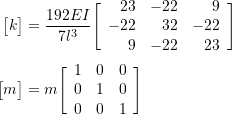### Approximate Methods for Multiple Degree of Freedom Systems: Rayleigh’s Quotient

For a conservative MDOF system, the total mechanical energy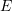is a constant so that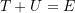or using the representations in (9.1) and (9.2)

(9.3)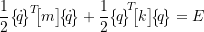Now consider the system vibrating in one of its normal modes at a natural frequency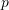. The amplitudes of the coordinates are given by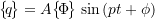where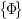is the corresponding mode shape and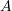is a constant. The derivatives of the coordinates will therefore be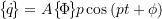The coordinates will have maximum values given by

(9.4)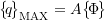which all occur simultaneously (when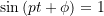). At the same instant,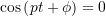so the derivatives of the coordinates will all be zero (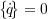).

Similarly the coordinate derivatives will have maximum values given by

(9.5)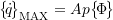when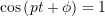. At this instant,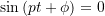so the coordinates themselves are all zero (i.e. the system is passing through its equilibrium configuration).

In summary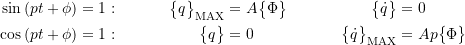Now, consider equation (9.3) at the instant when. We see that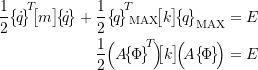or

(9.6)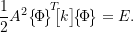Similarly, whenwe find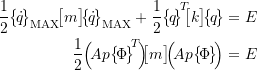or

(9.7)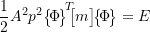Since the total energyis a constant for this conservative system, comparing equations (9.6) and (9.7 ) leads to the conclusion that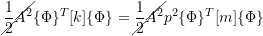or

(9.8)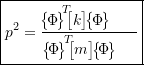Equation (9.8) is known as Rayleigh’s Quotient. This result tells us that if a mode shape corresponding to a particular natural frequency is used in the ratio in (9.8), then the result will be the associated natural frequency squared.

By itself this is not a particularly useful result since typically, knowing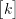and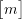for a particular system, we must findas part of the process of determining the mode shapes in the first place. However, equation (9.8) can be useful for obtaining approximate natural frequencies for MDOF systems. In essence, what we do is estimate a mode shape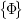for the system and Rayleigh’s Quotient returns an associated estimate of the natural frequency. Obviously, the choice of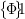will influence the value of the returned natural frequency, however it can be shown that if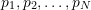are the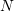natural frequencies on andegree of freedom system, then for any assumed mode shapethe frequencyreturned by Rayleigh’s Quotient satisfies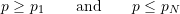i.e. the frequency returned by Rayleigh’s Quotient will always be higher than the lowest natural frequency and lower than the highest natural frequency in the system. Typically we are more concerned with the lowest (fundamental) frequency in a system, for which Rayleigh’s Quotient establishes an upper bound. (This frequency also provides a lower bound on the highest natural frequency of a system but this is usually of less interest.)

Remarkably good estimates of the fundamental natural frequency can be obtained even if the assumed mode shape(also called a trial vector) is not equal to the actual first mode shape.

#### EXAMPLE

(a) For the system shown above, estimate the fundamental natural frequency using Rayleigh’s Quotient. Assume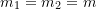and use trial vectors of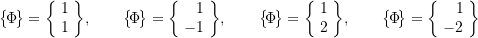and comment on the results. It was previously determined that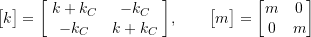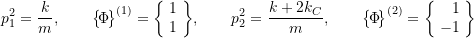(b) Repeat the calculations in part (a) assuming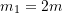,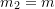, and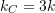.

Note that the exact solution in this case is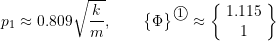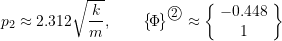#### EXAMPLE

Use Rayleigh’s method to estimate the fundamental natural frequency for a light simply supported beam supporting three equally spaced identical masses.

Assume trial vectors of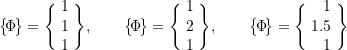Note that for this problem